# An Elementary Course on Turbocompressors

## 2.1 Introduction

When preparing ourselves to make the analysis of a compression system, an acknowledgement of the gas nature is of fundamental importance.

The gas nature comprises three aspects related to the gas characteristics that summarises appropriately its influence upon turbocompressors performance. They are:

• Gas Composition
• Gas Equation of State
• Gas Compressibility

In this lesson of our course we will try to explain the manner in which these aspects of gas behavior should be taken into account during compression calculations.

## 2.2 Gas Composition

The overwhelming majority of gases manipulated in process industry are mixtures of substances rather than pure substances. Fortunately, gas mixtures are completely homogeneous, allowing us to consider an resulting average behavior and then to proceed in the same way as if dealing with pure substances. In any case, it's impossible to deny that the calculations of gas mixtures properties involve some specific difficulties.

The problems appear still before the starting of the calculations. The gas composition is obtained by sampling followed by laboratory analysis and the tasks involved must be executed very carefully, because there is a large sensitivity of the results to the errors eventually made during these activities.

Mixtures compositions are almost always expressed by means of sets of data representing molar concentration (or molar fraction) of each included molecular species.

Let's think about mol as an equivalence to a certain amount of mass. For a substance exhibiting a known molecular weight (MW), its mass (m) corresponds to the following number of mols (n):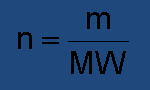(2.01)

The concept of mol doesn’t mean too much for those people interested in compressors subject. We are going to get rid of it as soon as possible, using mass instead. At the moment, we need to learn how to manipulate molar fractions, because this is the best way to reach gas composition.

Molar fraction (y) is the ratio between the number of moles of a given species and the total number of moles existing in the mixture. For a generic component “i”:(2.02)

Obviously, the sum of the molar fractions of all components of the mixture is equal to 1.0 or 100%:(2.03)

Since the number of moles is the amount of mass of the substance divided by its molecular weight, we can easily find a relationship between the molar fractions and the mass of each component in the mixture: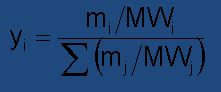(2.04)

A gas mixture can also be considered as having an apparent molecular weight equivalent to the weighted average of individual molecular weights with respect to each component molar fraction: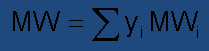(2.05)

This formula is extremely important because the molecular weight is a parameter associated to gas composition with a straight influence on turbocompressors performance.

Application Example:Suppose that 32 grams of methane (MW=16) and 14 grams of nitrogen (MW=28) form a gaseous mixture. Our duty is to find the respective molar fractions and the molecular weight of the gas.There are two other properties of mixtures frequently needed for thermodynamic calculations. They are critical pressure (Pc) and critical temperature (Tc). The critical properties concept comes from the limitation of the liquid-vapor saturation line observed in phase diagrams of pure substances.

The definition of a critical state for mixtures is complicated specially because there is no correspondence between pressure and temperature during changes of phase, as in pure substances. The critical state of a mixture is defined by the pair (pressure, temperature) in which the difference of liquid and vapor densities finally disappear, giving raise to a unique phase.

For implementing simplified engineering calculations we generally use heuristic rules. The most common procedure is to consider the averaged values of either the critical pressure and the critical temperature of the mixture, weighted in function of the respectives molar fractions:(2.06)

These quantities are more often called pseudo-critical pressure and pseudo-critical temperature, perhaps to emphasise that they are product of the experimental evidence.

Also usual is to calculate the critical temperature with the above formula and then to determine the critical pressure by means a relationship involving the critical compressibility factor and the critical specific volume of each component: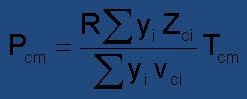(2.07)

## 2.3 Equations of State

There is a very important thermodynamic principle, named State Postulate, which can be shortly enunciated in the following way:

“The state of a gas is fully defined if two of its thermodynamic properties are known, for instance pressure and temperature, besides its chemical composition. Under these conditions, any other gas property has a unique value that can be obtained by means of charts, tables or equations”.

A very useful application of this principle consists in the determination of the specific volume (or density) of a gas stream. Even though in general sense specific volume is a measurable quantity, this is not applicable to moving fluids. In this case we are forced to measure pressure and temperature to find the specific volume by means of any kind of expression of the P-v-T behavior of the gas: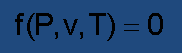(2.08)

Many implementations of such correlation has been developed since Thermodynamics was established as a formal science. The Perfect Gas Equation is the simplest of all equations of state and refers to a hypothetic gas behavior described by: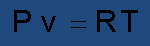(2.09)

where:

• P = absolute pressure
• T = absolute temperature
• V = specific volume
• R = particular gas constant

No real gas behaves exactly in compliance with this model, but it gives acceptable results when applied to gases submitted to low pressures and not very low temperatures.

The expression “ideal gas” is frequently employed in place of “perfect gas”, but we prefer not to do that, in order to avoid confusion with “ideal process”, which is an important concept in compression theory.

The usefulness of the perfect gas assumption goes far beyond providing means to evaluate gas density. Its simplicity makes possible the attainment of closed analytical solutions for many important thermodynamic calculations. Moreover, this model offers great praticality when used to address qualitative aspects of thermodynamic phenomena.

The P-v-T relationships of distinct perfect gases differ only by the constant R. It can be found dividing the so-called Universal Gas Constant (Ru) by the molecular weight (MW) of the particular gas we are dealing with. Adopting SI units:(2.10)

Frequently we are faced to practical situations in which the gas exhibits strong ideality deviation, and the use of more advanced gas models becomes mandatory, although they require numerical solutions. Thus, for the sake of accuracy, we are forced to abandon all the conveniences of the perfect gas model. In these cases, we have to choose among around a half dozen of real gas equations generally used in ordinary engineering studies, the one that best fit the intended application.

Most of these equations can be expressed analyticaly as a f(P, v, T)=0 relationship. Nonetheless, they are more commonly written in the form: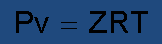(2.11)

Here Z, named Compressibility Factor, acts as a corrective parameter applied to the perfect gas equation. Wether this parameter is higher or lower than the unity, it indicates some deviation from ideality.

Note that Z is not a constant, but a thermodynamic property just like P, v or T. Therefore it can be represented by a pair of two other known properties, let’s say P and T, for instance: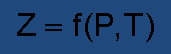(2.12)

We don’t want to go further in this matter right now, but perhaps it is worth presenting here the one considered the easiest-to-use real gas state equation that shows yet good precision in many practical cases. We are talking about the Redlich-Kwong equation of state :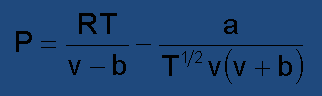(2.13)

In comparison to perfect gas equation, we realize a meaningful increase in complexity. This is what we have to pay for the improvement in accuracy, accomplished by the introduction of two corrective constants a and b. These constants must be specifically calculated for each gas: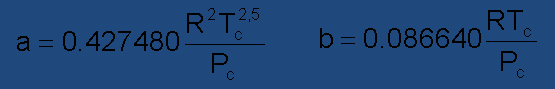(2.14)

The Redlich-Kwong equation can also be written as a polynomial function of the compressibility factor, resulting in: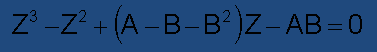(2.15)

The parameters A and B are dependent on pressure, temperature and gas composition (molecular weight and critical properties):(2.16)

The solution of this third degree polynomial equation is known, but its explanation is beyond the scope of our course. After all, is not difficult to find out a short computer program or an Excel spreadsheet dedicated to this subject. As last resort, we suggest the solution by the Newton-Raphson approach, consisting in the iterative calculation of the Z factor based on the following relationship:(2.17)

In our case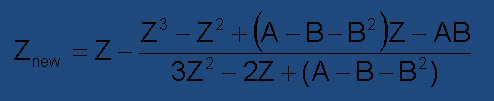(2.18)

As an initial guess, enter Z=1.0 into the right side member of the above equation to obtain a new value for this parameter. Repeat sucessively this procedure until you get a satisfactorily small difference between the Z value entered in the equation and the result of the calculation.

Application Example: Methane is submitted to 80.0 bar of pressure and 300.0 K of temperature. Try to find its compressibility factor under this condition, using the Redlich-Kwong equation. Supplied data for Methane:

• Molecular weight: 16.043
• Critical pressure: 46.0 bar
• Critical temperature: 190.4 K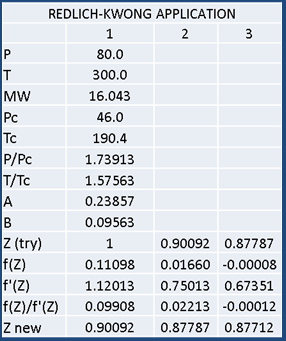Solution:

The calculations are tabulated here, each column corresponding to an iteration. Three attempts lead us to a compressibility factor of 0.87712, very close to the correct value, which is 0.8799.

The molecular weight of the gas was not necessary because Redlich-Kwong is a two-parameters equation dependant only on critical pressure and temperature.

The Redlich-Kwong equation is in the limit of the solvability by analytical means. Almost all the equations that appeared thereafter require numerical solution. In the past this was a substantial drawback, but nowadays the computational approach is the only way for solving problems in sciences or engineering.

Now we gonna review some additional features of the perfect gases. As we told earlier, the perfect gas model brings us interesting facilities. Some of them derive from the fact that the specific heat capacities of a perfect gas are properties exclusively dependant on temperature. Additionally, in Termodynamics books we learn that specific heat capacity at constant pressure is well represented by a smooth polynomial function of temperature: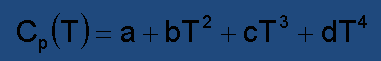(2.19)

For finding specific heat capacity at constant volume, just make (perfect gas only):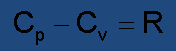(2.20)

Moreover, the enthalpy variation of a gas moving from a state 1 to a state 2 is an information often needed for use in compression analysis. When dealing with perfect gases, this quantity can be found simply by means of: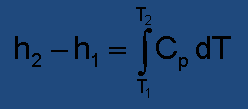(2.21)

Consequently: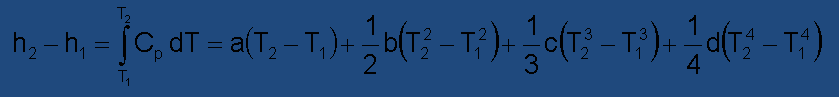(2.22)

Note: For gas mixtures, use the weighted average of each specific heat capacity coefficient based on the molar fractions of the components.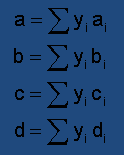(2.23)

Application Example: Methane gas, initially at a pressure of 80 bar and temperature of 27°C, is compressed by a turbocompressor until being discharged at 200 bar and 127°C. Let’s find the increase of the gas enthalpy based on perfect gas behavior.

The Cp of Methane in low density conditions, measured in kJ/(kg.K), is given by the equation: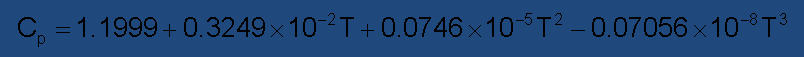where T is the temperature in Kelvin degrees. Taking the extreme temperatures of our compression process, respectively T1=300 K and T2=400 K, and entering into the enthalpy formula here presented, we get: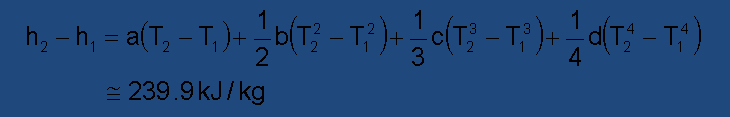Obviously, the coefficients a, b, c, d used were taken from methane heat capacity equation.

The true value experimentally obtained is 224.10 kJ/kg, showing an error of about 7%. This was expected because the perfect gas model does not work properly for methane at these pressure levels.

## 2.4 Gas Compressibility

The industrial compressors, and why not to say specially the turbocompressors, show performance strongly affected by the compressibility of the gas.

Think about what happens in liquid pumps applications. Except in case of a pump designed for very high pressures, the fluid goes from the suction to the discharge maintaining practically the same density. This fact makes easier the choice of the proper geometry of the equipment targeting the attainment of the best flow conditions.

With regard to compressors, the situation is very different. The gas density changes progressively as the pressure is augmented along the machine. The temperature is also variable. It’s imperative to predict these unavoidable effects, in order to obtain a good design.

In reason of the intense use of the term compressibility in thermodynamic studies, we ought to explain the exact meaning we intend to give to this word. Let start considering that a certain amount of a gaseous substance is contained in a cylinder-and-piston device. Means for measurement of gas pressure and specific volume are provided.

Then, from an initial condition (P1, T1, v1,..) the gas is slowly compressed by pressing down the movable piston, until another arbitrary condition (P2, T2, v2,..) is reached.

The most obvious index for evaluating the gas compressibility manifested in such process is given by the ratio between the change of pressure imposed to the gas and the corresponding change of specific volume:(2.24)

The lower this ratio, the higher the gas compressibility, because less pressure should be applied to produce a given volume contraction. Inversely, a high value of this ratio would indicate a low gas compressibility.

In order to obtain a non-dimensional quantity with the same purpose, we have to measure either pressure and volume changes on relative basis:(2.25)

A minus signal is conveniently adopted because otherwise a negative value would always result, when referring to compression processes.

Now we have to go a little deeper into Thermodynamics and say that we need to impose two restrictions to our imaginary experience, if we want it to produce meaningful and repetitive results:

1. We have to make some hypothesis about the heat exchanged during the process, and the easiest way to do that is to suppose the compression to be adiabatic (no heat exchanged).

2. We have to assume a compression carried out along an infinitesimally slow path, with the purpose of avoiding entropy generation, consequently affecting the results of the experience.

In harmony with the second assumption we would do better considering an infinitesimal amplitude for the change of state promoted by our experience. Thus we get:(2.26)

Remember that the subscript “s” in the formula stands for “at constant entropy”.

Adiabatic Compressibility Coefficient (k) is what we chose to call this parameter. It’s a thermodynamic property of the gas, measured at a given point belonging to an isoentropic compression. In practice, this valuable coefficient makes feasible the analytical treatment of compression problems, even though some slight simplification is always necessary to reach the solutions. Numerical approaches would be the the other option.

Numerical approaches for solving mathematical problems show a strong drawback when compared to analytical procedures. The influence of the parameters involved in the problem does not appear clearly to the analist. A laborious evaluation of the model sensibility is the only way to access this information.

Fortunately, in many applications of low density gases, it’s reasonable to suppose the invariability of the coefficient k over the entire range of thermodynamic states lying on the gas compression path. In this case, a closed algebraic function in P and v results from the integration of the expression above:(2.27)

That’s why the parameter k is also called “adiabatic exponent” or “isoentropic exponent”.

The above expression is known as the adiabatic compression equation of perfect gases, because the low density is a marked characteristic of the gases which behaves like perfect gases. It’s usually representated in a Pxv diagram, as shown below.

The constant that appears on the right side of the adiabatic formula has a pure mathematical sense, not physical . It’s the constant that results ordinarilly from the integration of a differential equation. In turn, the exponent k is a property of the compressed gas.

Indeed, the equation(2.28)

represents an infinite number of curves having the same value for k. These relationships are distinguished by the service conditions and can be written in a more concrete form: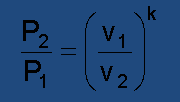(2.29)

Using general thermodynamic relationships, the definition of the adiabatic compressibility coefficient can be changed into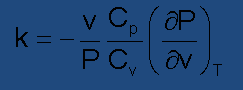(2.30)

Some interesting conclusions arise when we combine this formulation with the hypothesis of perfect gas. Considering the Pv=RT correlation to solve the partial derivative above, we get: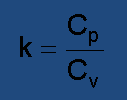(2.31)

As we see, k is equivalent to the ratio of the gas specific heat capacities respectively at constant pressure (Cp) and at constant volume (Cv). However, we know that the difference between the heat capacities of a perfect gas corresponds to its particular constant R, so the rate of these numbers could not be also a constant, except if they were constants themselves. But actually they are dependent on the gas temperature.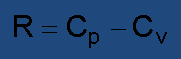(2.32)

The conclusion is that, rigorously speaking, the adiabatic exponents k of perfect gases always changes with temperature, in such way that the acceptance of its invariability is just an attempt to make things easier.

Should we get disappointed because of that? Not at all.

Look at the table shown here and see the expected values of the adiabatic exponents of common gases, taken in two different temperatures, under pressures sufficiently low to justify the admissibility of the perfect gas supposition. For the majority of purposes, the variations can be neglected.

Insofar as the pressure (and density) increases, the compliance with the invariability assumption gets worse.

Combining the above relationships: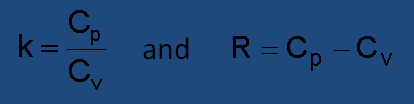(2.33)

we get: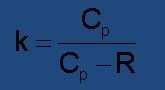(2.34)

This is a very interesting formula for finding the parameter k constant relative to a perfect gas process, because the data on specific heat capacity are much more common in technical literature. We recommend to take the two extreme temperatures in the process (T1 and T2) to calculate k1 and k2 and subsequently the averaged k:(2.35)

Application Example:Methane gas initially at 80 bar of pressure and 27°C of temperature is compressed by a turbocompressor, being discharged at 200 bar and 127°C. Find the averaged exponent k related to this process, assuming perfect gas behavior.

The molecular weight of the methane is 16.043. The Cp of Methane in low density conditions , measured in kJ/(kg.K) is given by the equationwhere T is the temperature in Kelvin degrees.

Solution: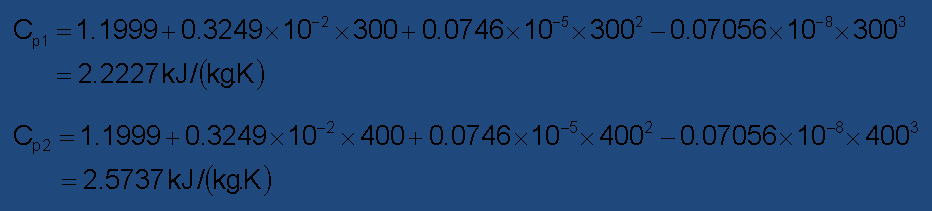That’s All.We got k=1.2781 as an hypotetically constant adiabatic exponent applicable along our process.

Still regarding the compression of perfect gases, it’s possible to know what happens to the temperature while the gas is compressed adiabatically and slowly. Starting with the relationship between pressure and specific volume:(2.36)

and combining with the perfect gas equation applied to 1 and 2: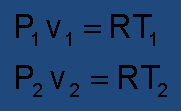(2.37)

we get, solving for (P,T) and for (V,T) respectively: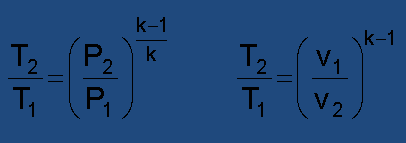(2.38)

These results show that gases having larger values of adiabatic exponent tend to produce higher discharge temperatures and lower volume contractions when compressed.